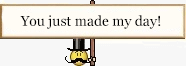# Statics: Force Analysis of a Pipe Bender Machine

## Homework Statement

.

The pipe bender consists of two grooved pulleys mounted and free to turn on a fixed frame. The pipe is bent into the shape shown by a force P=300N.Calculate the forces supported by the bearings of the pulleys.
[/B]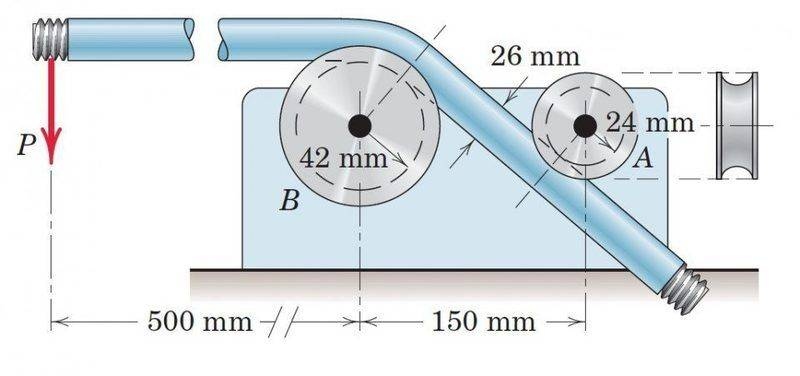## Homework Equations

The force equilibrium equations in two mutually perpendicular directions x- and y, i.e, ∑Fx=0 and ∑Fy=0 (treating the problem as two-
dimensional)

The moment equilibrium equation about any point O on or off the body i.e,[/B] ∑MO=0.

3. The Attempt at a Solution

My confusion is whether to neglect the frictional forces on the pipe by the two pulleys which is essential to drive the pipe between the pulleys.If we consider the frictional forces between the pulleys A and B and the pipe surface then the problem becomes statically indeterminate as we would have four unknowns which are,NA and NB - the normal reactions by pulleys A and B on the pipe and FA and FB - the frictional forces exerted by the pulleys A and B on the pipe with only three independent equations of equilibrium in hand.

On the other hand if we neglect the frictional forces assuming the pipe and pulley surfaces to be perfectly smooth we would have only two unknowns and the problem can be solved theoretically.But again we need to resolve the force P into components along and perpendicular to the pipe axis(the portion of pipe between the pulleys) for writing the force equilibrium equations.So I need to calculate the angle between the line of action of P and the pipe axis which I am unable to do with the given data in hand.

Can someone help?

Nidum
Gold Member
The problem is about the forces acting after the bar has already been bent to shape .

Clue : The pulleys are free to rotate .

Can you see how to proceed with solution ?

•Soumalya
Nidum
Gold Member
PS: There is enough information given in the problem to work out the bend angle .

•Soumalya
haruspex
Homework Helper
Gold Member
which is essential to drive the pipe between the pulleys.
As I read it, the pipe is not passing through the pulley system. The bending comes from the force P applied at right angles to the pipe. The pulleys merely act as fulcrum and load.
what can you say about the directions and lines of action of the forces exerted by A and B on the pipe?

•Soumalya
The problem is about the forces acting after the bar has already been bent to shape .

Since it is asked to determine the reactions at the bearings of the pulleys I assume the entire pipe length as shown in the figure(including the portion of the pipe between the pulleys and at the the point of application of force P)should be included in a free body diagram, so that we may be able to determine the contact forces on the pipe by the pulleys(and hence the equal and opposite contact forces by the pipe on the pulleys).Please correct me if am I am wrong.

Clue : The pulleys are free to rotate .

Can you see how to proceed with solution ?

From the clue the only conclusion I can perceive is that there are no reactive couples acting on the pulleys by the bearings supporting them.

Did I miss something here?

Can you see how to proceed with solution ?

Noting haruspex"s comment about the pulley A acting as a fulcrum and the reactive force on the pipe by pulley B as a load and the fact that the problem doesn't mention the pipe 'passing' through the pulleys(no relative motion between the pipe and pulley surfaces) there cannot be any frictional forces between the pipe and pulley surfaces.Hence the forces FA and FB as previously assumed doesn't exist and we have only two unknown forces NAand NB as the normal reactions to the pipe by the pulleys A and B respectively.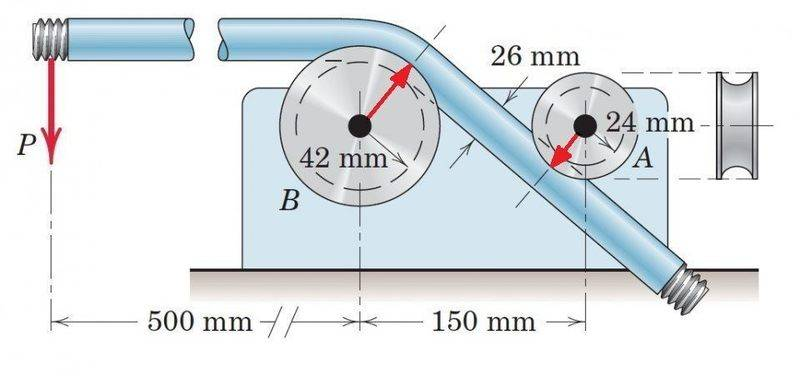Am I moving in the right direction?

PS: There is enough information given in the problem to work out the bend angle .

I still can't figure out how to calculate the bend angle.Can you provide a geometric sketch that can help?

As I read it, the pipe is not passing through the pulley system. The bending comes from the force P applied at right angles to the pipe. The pulleys merely act as fulcrum and load.
what can you say about the directions and lines of action of the forces exerted by A and B on the pipe?

Noting your hints carefully I realized that the frictional forces FA and FB by the pulleys A and B respectively on the pipe as previously assumed cannot exist.Then we must have only two normal reactive forces NA and NB on the pipe by pulleys A and B respectively.The lines of action of these forces pass through the points of contact between the pulleys and pipe and normal to the tangent to the surfaces at the points of contact.The directions of these forces should be away from the pulleys towards the pipe along their respective lines of action.

#### Attachments

haruspex
Homework Helper
Gold Member
Since it is asked to determine the reactions at the bearings of the pulleys I assume the entire pipe length as shown in the figure(including the portion of the pipe between the pulleys and at the the point of application of force P)should be included in a free body diagram, so that we may be able to determine the contact forces on the pipe by the pulleys(and hence the equal and opposite contact forces by the pipe on the pulleys).Please correct me if am I am wrong.

From the clue the only conclusion I can perceive is that there are no reactive couples acting on the pulleys by the bearings supporting them.

Did I miss something here?

Noting haruspex"s comment about the pulley A acting as a fulcrum and the reactive force on the pipe by pulley B as a load and the fact that the problem doesn't mention the pipe 'passing' through the pulleys(no relative motion between the pipe and pulley surfaces) there cannot be any frictional forces between the pipe and pulley surfaces.Hence the forces FA and FB as previously assumed doesn't exist and we have only two unknown forces NAand NB as the normal reactions to the pipe by the pulleys A and B respectively.
View attachment 102656

Am I moving in the right direction?

I still can't figure out how to calculate the bend angle.Can you provide a geometric sketch that can help?
Closer, but take a look at the three forces you have drawn. Can this represent static equilibrium? You have made a wrong assumption about one of them.

•Soumalya
Closer, but take a look at the three forces you have drawn. Can this represent static equilibrium? You have made a wrong assumption about one of them.

It seems I am definitely wrong somewhere because according to my assumption the pipe is a three force member(the three forces are not parallel to each other)under equilibrium and the lines of action of the three forces must have been concurrent.Either or both the assumed lines of action of the forces exerted by the pulleys on the pipe must be wrong.But I am clueless as to what should the correct reactions by the pulleys on the pipe.

haruspex
Homework Helper
Gold Member
It seems I am definitely wrong somewhere because according to my assumption the pipe is a three force member(the three forces are not parallel to each other)under equilibrium and the lines of action of the three forces must have been concurrent.Either or both the assumed lines of action of the forces exerted by the pulleys on the pipe must be wrong.But I am clueless as to what should the correct reactions by the pulleys on the pipe.
As you noted, the forces exerted by the pulleys on the pipe must be normal to the contacting surfaces. In the case of pulley A there is a single point of contact, so there is no difficulty in finding the normal. But for pulley B, there is an arc of contact. This leaves a range of possibilities for the direction of the 'normal'. How can you decide the direction?

•Soumalya
As you noted, the forces exerted by the pulleys on the pipe must be normal to the contacting surfaces. In the case of pulley A there is a single point of contact, so there is no difficulty in finding the normal. But for pulley B, there is an arc of contact. This leaves a range of possibilities for the direction of the 'normal'. How can you decide the direction?

Considering that there are only three forces acting on the pipe in equilibrium namely P,NA and NB and the three forces do not form a parallel force system,the lines of action of the three forces must be concurrent.Since the directions of both P and NA are fixed and can be perceived clearly the direction of the line of action of the force NB is easily determined from the fact that it must pass through the point of concurrency of the forces P and NA.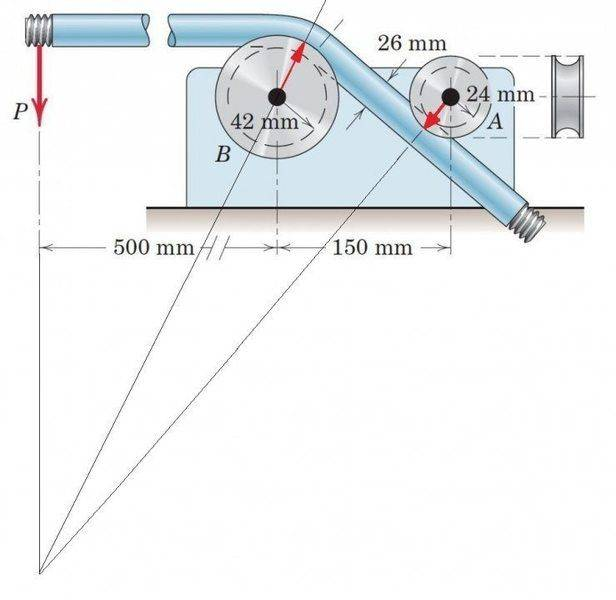To determine analytically the direction of the line of action of NB we may assume that NB makes an angle of θ with the vertical.Since the direction of NA can be determined we would have three unknowns in the problem which are NA,NB and θ.They can be determined using the three independent equations of equilibrium.

But the problem is I am unable to calculate the bend angle.Is there sufficient data provided to be able to calculate the bend angle?

If yes can you provide a geometric sketch to illustrate?

haruspex
Homework Helper
Gold Member
Is there sufficient data provided to be able to calculate the bend angle?
Yes.
Draw a line from A's centre parallel to the pipe there. Drop a perpendicular to it from B to meet it at C.
Concentrate on triangle ABC.

•Soumalya
Yes.
Draw a line from A's centre parallel to the pipe there. Drop a perpendicular to it from B to meet it at C.
Concentrate on triangle ABC.
I was being stupid here.That was a relatively simple task!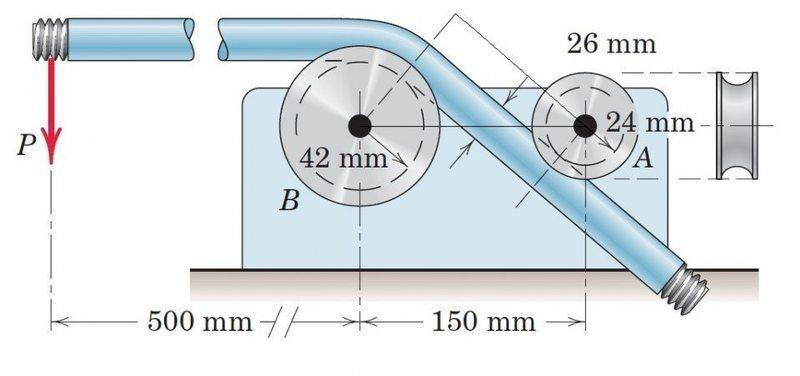You are so helpful Sir haruspex